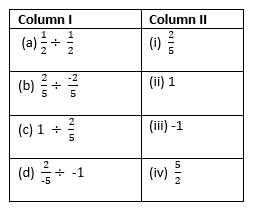# Rational numbers worksheet for Class 7

## True or false

Question 1
(a) Every natural number is a rational number but every rational number needs not be a natural number
(b) Zero is a rational number.
(c) Every rational number is a whole number.
(d) Two rational numbers with difference numerators can't be equal.
(e) Every fraction is a rational number.
(f) Sum of two rational numbers is always a rational number.
(g) The rational number $\frac {-3}{5}$ lies to the right of zero on the number line.
(h) Every natural number is a rational number but every rational number need not be a natural number.
(i) $\frac {2}{4}$ is equivalent to $\frac {4}{8}$
(j) The rational numbers $\frac {-11}{-12}$ and $\frac {-7}{8}$ are on the opposite sides of zero on the number line

(a) T
(b) T
(c) F
(d) F
(e) T
(f) T
(g) F
(h) T
(i) T
(j) T

## Fill in the blanks

Question 2.
(a) A rational number $\frac{p}{q}$ is said to be in the lowest form if p and q have no __________
(b) The rational number $\frac {- 12}{-17}$ Lies to the ____________ of zero on the number line.
(c) If $\frac{p}{q}$ is a rational number, then q can't be ___________
(d) Two rational numbers with different numerators are equal, if their numerators are in the same ______________ as their denominators.
(e) Two rational numbers are equal if they have the same __________ form.
(f) A rational number $\frac{p}{q}$ is negative if p & q are of __________ sign.
(g) If the product of two non-zero rational number is 1, then they are ______________ of each other.
(h) Between any two distinct rational numbers there are ___________ rational numbers.
(i) Additive inverse of $\frac {2}{3}$ is ______.
(j) The reciprocal of ______ does not exist.

(a) common factor
(b) right
(c) 0
(d) ratio
(e) simple
(f) oppossite
(g) reciprocal
(h) infinite
(i) $\frac {-2}{3}$
(j) zero

## Numerical questions

Question 3.
By what number should we multiply $\frac {-8}{15}$ , so that the product may be 24.

$\frac {-8}{15} \times 24= -45$

Question 4.
What should be subtracted from $\frac {-3}{4}$ so as to get $\frac{5}{9}$ ?

$\frac {-3}{4} - \frac{5}{9}= \frac { -3 \times 9 - 4 \times 5}{36}= \frac {-47}{36}$

Question 5.
Subtract $\frac {-3}{8}$ from $\frac {-5}{7}$

$\frac {-5}{7} - \frac {-3}{8}= \frac {-5 \times 8 + 3 \times 7}{56}=\frac {-19}{56}$

Question 6.
The cost of $4\frac{1}{2}$ meters of cloth is Rs. $85\frac{1}{2}$ find the cost of one meter cloth.

$85\frac{1}{2} \div 4\frac{1}{2}= \frac {170}{2} \div \frac {9}{2} = \frac {170}{2} \times \frac {2}{9} = \frac {170}{9} = 18 \frac {8}{9}$

Question 7.
Simplify $(\frac {-5}{8} \times \frac {3}{7} \times \frac {4}{-15})+(\frac {4}{7} \times \frac {-21}{8})$.

$(\frac {-5}{8} \times \frac {3}{7} \times \frac {4}{-15})+(\frac {4}{7} \times \frac {-21}{8})= \frac {1}{14} + \frac {-3}{2} = \frac {-20}{14} = \frac {-10}{7}$

Question 8.
A stairway consists of 14 stairs, each $32\frac{5}{7}$ cm high. What is the vertical height of the stairways ?

$14 \times 32\frac{5}{7}= 14 \times \frac {224}{7} = 448$ cm

Question 9.
Arrange the rational numbers $\frac {-7}{10}$,$\frac {5}{-8}$,$\frac {2}{-3}$ in the ascending order.

Converting them into same denominator by using LCM

$\frac {-7}{10}= \frac {-84}{120}$
$\frac {5}{-8}=\frac {-75}{120}$
$\frac {2}{-3}= \frac {-80}{120}$

So Ascending order will be
$\frac {-84}{120}$ < \frac {-80}{120} < \frac {-75}{120}\frac {-7}{10} < \frac {2}{-3} < \frac {5}{-8}$## Multiple Choice Questions Question 10. Which of the following rational numbers is equal to its reciprocal? (a) 1 (b) 2 (c)$\frac {1}{2}$(d) 0 Question 11. Which is greater number in the following: (a)$\frac {-1}{5}$(b) 0 (c)$\frac {1}{5}$(d) -5 Question 12. Which is lowest number in the following: (a)$\frac {-1}{2}$(b) 0 (c)$\frac {1}{2}\$
(d) -2
Question 13.
Match the Column(a) a -> ii , b -> iii , c -> iv , d -> i
(b) b -> ii , a -> iii , c -> iv , d -> i
(c) a -> ii , b -> iii , c -> i , d -> iv
(d) a -> i , b -> iii , c -> iv , d -> ii
Question 14.
To reduce a rational number to its standard form, we divide its numerator and denominator by their
(a) LCM
(b) HCF
(c) product
(d) multiple

10.(a)
11. (c)
12. (d)
13.(a)
14.(b)